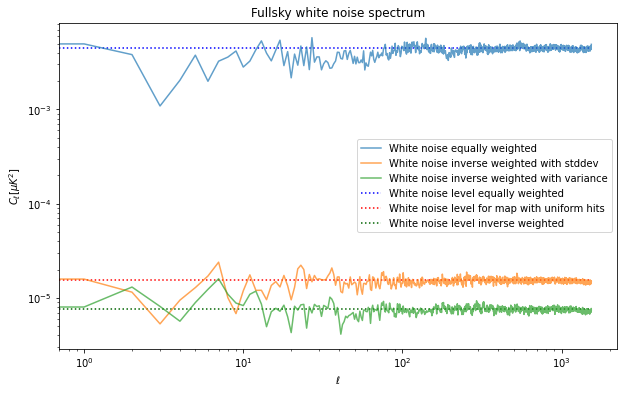# Handle white noise with healpy 3 not-uniform and partial sky coverage

Simulate white noise maps and use hitmaps
cosmology
python
healpy
Published

June 21, 2020

import healpy as hp
import numpy as np
%matplotlib inline
import matplotlib.pyplot as plt
import astropy.units as u
hp.disable_warnings()

In this third notebook, we will handle a case of not-uniform and partial sky coverage.

# Number based on Simons Observatory SAT UHF1 array of detectors

net = 10. * u.Unit("uK * sqrt(s)")

5 years with a efficiency of 20%:

integration_time_total = 5 * u.year * .2

hitmap_url = "https://portal.nersc.gov/project/sobs/so_mapsims_data/v0.2/healpix/ST0_UHF1_01_of_20.nominal_telescope_all_time_all_hmap.fits.gz"
!wget $hitmap_url --2020-12-11 10:35:42-- https://portal.nersc.gov/project/sobs/so_mapsims_data/v0.2/healpix/ST0_UHF1_01_of_20.nominal_telescope_all_time_all_hmap.fits.gz Resolving portal.nersc.gov (portal.nersc.gov)... 128.55.206.24, 128.55.206.26 Connecting to portal.nersc.gov (portal.nersc.gov)|128.55.206.24|:443... connected. HTTP request sent, awaiting response... 200 OK Length: 3212515 (3.1M) [application/x-gzip] Saving to: ‘ST0_UHF1_01_of_20.nominal_telescope_all_time_all_hmap.fits.gz.10’ ST0_UHF1_01_of_20.n 100%[===================>] 3.06M --.-KB/s in 0.1s 2020-12-11 10:35:43 (30.0 MB/s) - ‘ST0_UHF1_01_of_20.nominal_telescope_all_time_all_hmap.fits.gz.10’ saved [3212515/3212515]  hitmap = hp.read_map("ST0_UHF1_01_of_20.nominal_telescope_all_time_all_hmap.fits.gz") hitmap /= hitmap.max() hp.mollview(hitmap)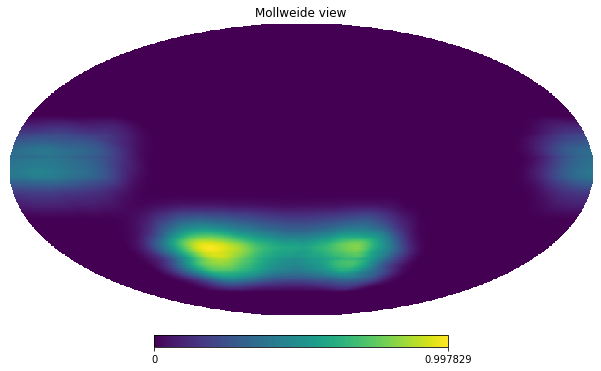## Generic partial sky survey We have now a sky coverage which not uniform nside = 512 npix = hp.nside2npix(nside) hitmap = hitmap / hitmap.sum() * integration_time_total.to(u.s) hitmap_plot = hitmap.value.copy() hitmap_plot[hitmap == 0] = hp.UNSEEN hp.mollview(hitmap_plot, unit=hitmap.unit)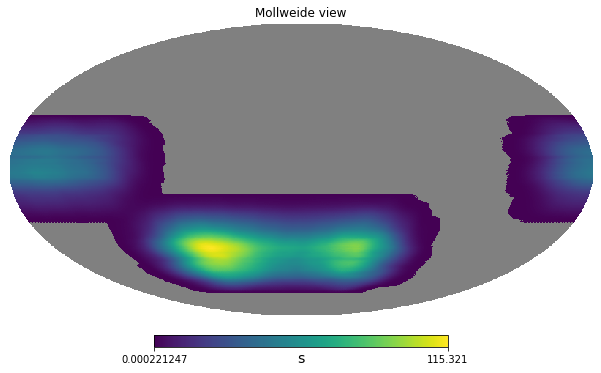variance_per_pixel = \ (net**2 / hitmap).decompose() /home/zonca/zonca/p/software/healpy/healpyvenv/lib/python3.7/site-packages/astropy/units/quantity.py:477: RuntimeWarning: divide by zero encountered in true_divide result = super().__array_ufunc__(function, method, *arrays, **kwargs) variance_per_pixel[np.isinf(variance_per_pixel)] = 0 m = np.random.normal(scale = np.sqrt(variance_per_pixel), size=len(variance_per_pixel)) * np.sqrt(variance_per_pixel).unit variance_per_pixel.max() $$4.51983 \times 10^{-7} \; \mathrm{K^{2}}$$ m.value[hitmap==0] = hp.UNSEEN m = m.to(u.uK) m.value[hitmap==0] = hp.UNSEEN hp.mollview(m, unit=m.unit, min=-10, max=10, title="White noise map")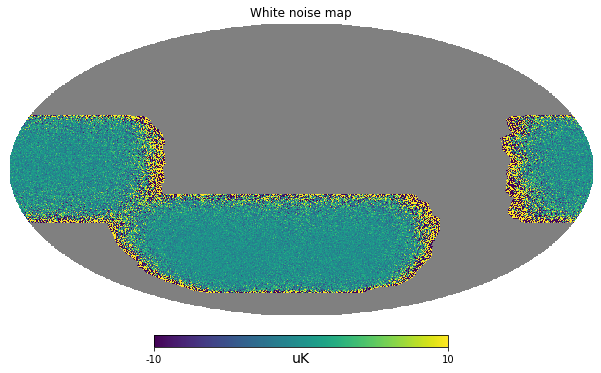## Power spectrum sky_fraction = hp.mask_good(m).sum()/len(m) cl = hp.anafast(m) / sky_fraction cl[100:].mean() 0.0044214096150382255 pixel_area = hp.nside2pixarea(nside) white_noise_cl = (variance_per_pixel[variance_per_pixel>0].mean() * pixel_area).to(u.uK**2) white_noise_cl_uniform = 1.5341266e-5 * u.uK**2 plt.figure(figsize=(6,4)) plt.loglog(cl, label="Map power spectrum", alpha=.7) plt.hlines(white_noise_cl.value, 0, len(cl), color="blue", label="White noise level") plt.hlines(white_noise_cl_uniform.value, 0, len(cl), label="White noise level uniform") plt.title("Fullsky white noise spectrum") plt.xlabel("$\ell$") plt.ylabel("$C_\ell [\mu K ^ 2]$");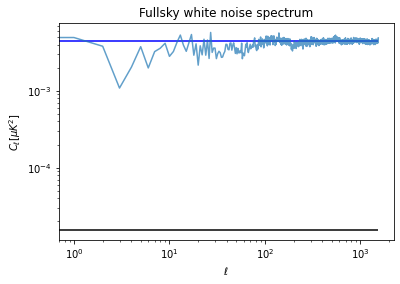sky_fraction 0.38526121775309247 ## Pixel weighting in the power spectrum When we have un-uniform noise across the map, it is advantageous to weight the pixels differently before taking the power spectrum in order to downweight the noisiest pixels. If we weight by the square root of the number of hits per pixels, then we are normalizing the standard deviation per pixel to be the same across all pixels, in fact we recover the same noise level we had when we were spreading the hits uniformly in the sky patch. However, the optimal is actually to weight by the inverse variance, which means to weight by the hitmap, to estimate the value expected for this we need to weight the variance by the square of the hitmap (variance is in power so weighting the map by a quantity is equivalent to weighting the variance by its square). cl_apodized = hp.anafast(m * np.sqrt(hitmap)) / np.mean(hitmap) cl_apodized_inv_variance = hp.anafast(m * hitmap) / np.mean(hitmap**2) cl_apodized_inv_variance[100:].mean() / white_noise_cl_uniform.value $$0.49056891 \; \mathrm{\frac{1}{s^{2}}}$$ white_noise_cl / white_noise_cl_uniform $$291.08959 \; \mathrm{}$$ white_noise_cl $$0.0044656828 \; \mathrm{\mu K^{2}}$$ white_noise_cl_uniform $$1.5341266 \times 10^{-5} \; \mathrm{\mu K^{2}}$$ np.average(variance_per_pixel, weights=hitmap) * pixel_area * 1e12 $$1.5341266 \times 10^{-5} \; \mathrm{K^{2}}$$ white_noise_cl_inv_variance = np.average(variance_per_pixel, weights=hitmap**2) * pixel_area * 1e12 plt.figure(figsize=(10,6)) plt.loglog(cl, label="White noise equally weighted", alpha=.7) plt.hlines(white_noise_cl.value, 0, len(cl), color="blue", ls=":", label="White noise level equally weighted") plt.loglog(cl_apodized, label="White noise inverse weighted with stddev", alpha=.7) plt.hlines(white_noise_cl_uniform.value, 0, len(cl), color="red", ls=":", label="White noise level for map with uniform hits") plt.loglog(cl_apodized_inv_variance, label="White noise inverse weighted with variance", alpha=.7) plt.hlines(white_noise_cl_inv_variance.value, 0, len(cl), color="darkgreen", ls=":", label="White noise level inverse weighted") plt.title("Fullsky white noise spectrum") plt.legend() plt.xlabel("$\ell$") plt.ylabel("$C_\ell [\mu K ^ 2]\$");